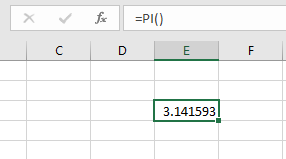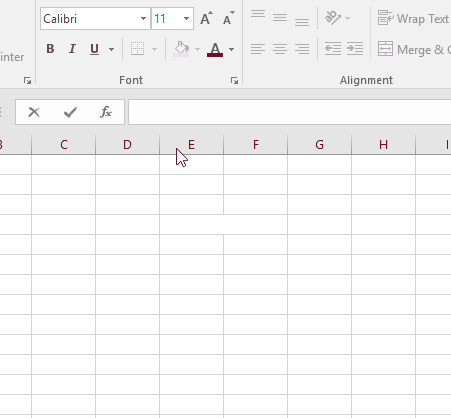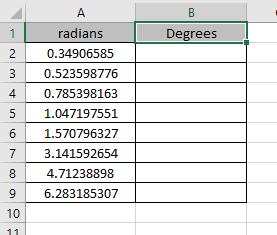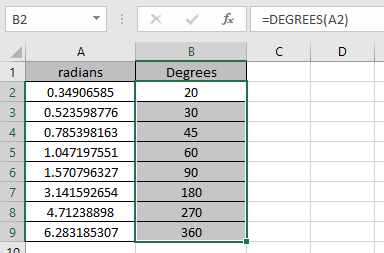# How to use the DEGREES Function in Excel

The radius of a circle is one-half of the distance across its center which makes an angle equal to one radian. A radian is approximate equals to 57.2958 degrees because a whole circle is 360 degrees and is equal to two pi radians.

1 degree = (?/ 180) = (3.14159/180) radians

The PI function in excel returns the value of which is approximately 3.14159...Now we will use the PI() function to get values from the DEGREES function in excel

The DEGREES function takes the value of the angle in radians and returns the angle in degrees.
Syntax:

=DEGREES (angle)Now let’s get this function via running it on some examples.Here we have some values as angle in radians and needs to get the angle in degrees
Use the formula:

=DEGREES(A2)As you can see from the above snapshot that angle in degrees can be extracted using the DEGREES function.

Hope you understood how to use DEGREES function and PI function in Excel. Explore more articles on Excel Date & Time functions here. Please feel free to state your query or feedback for the above article.

Related Articles:

How to use the RADIANS Function in Excel

Excel PI function

How to use SIN function in Excel

How to use the SINH Function in Excel

How to use the COSH Function in Excel

How to use the TANH Function in Excel

Popular Articles:

50 Excel Shortcuts to Increase Your Productivity

How to use the VLOOKUP Function in Excel

How to use the COUNTIF in Excel 2016

How to use the SUMIF Function in Excel

Terms and Conditions of use

The applications/code on this site are distributed as is and without warranties or liability. In no event shall the owner of the copyrights, or the authors of the applications/code be liable for any loss of profit, any problems or any damage resulting from the use or evaluation of the applications/code.